# Algebra II : Factorials

## Example Questions

### Example Question #1 : Multiplying And Dividing Factorials

Simplify: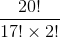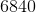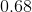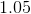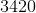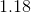Explanation:

Remember what a factorial is, and first write out what the original equation means. A factorial is a number that you multiply by all whole numbers that come before it until you reach one.

You can simplify because all terms in the expression 17! are found in 20!.

Thus: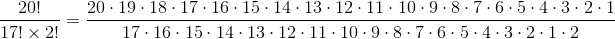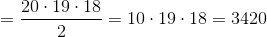### Example Question #2 : Multiplying And Dividing Factorials

Simplify: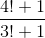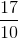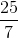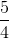Explanation:

Rewrite the factorials in multiplicative order.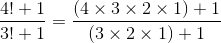In this scenario, the numbers of the factorial in the numerator and denominator CANNOT cancel.  Simplify by multiplying out the factorials.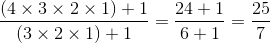### Example Question #11 : Multiplying And Dividing Factorials

Simplify the following expression: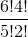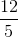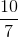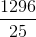Explanation:

n! indicates a factorial, which means the products of numbers from 1 to n.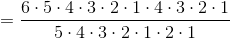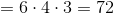### Example Question #12 : Multiplying And Dividing Factorials

Divide: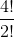Explanation:

Write out the terms for the factorial.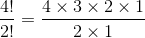Cancel out the common numbers in the numerator and denominator.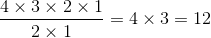The answer is.

### Example Question #13 : Multiplying And Dividing Factorials

Multiply: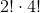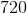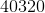Explanation:

To evaluate the product of these factorials, we will need to expand the factorials.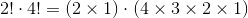Multiply all the values.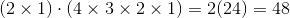The answer is:### Example Question #14 : Multiplying And Dividing Factorials

Divide: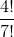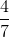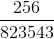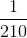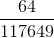Explanation:

Expand the factorials in the numerator and denominator.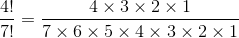The terms 1, 2, 3, and 4 can be eliminated from the numerator and denominator.  The simplification becomes: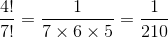### Example Question #15 : Multiplying And Dividing Factorials

Solve: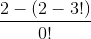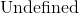Explanation:

Evaluate the factorials first.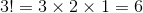Substitute the values back into the expression.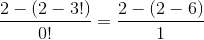Simplify the numerator.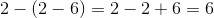### Example Question #16 : Multiplying And Dividing Factorials

Solve the factorial: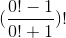Explanation:

Evaluate each factorial inside the parentheses.

Zero factorial is equal to.  Simplify by order of operations.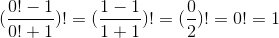The answer is.

### Example Question #17 : Multiplying And Dividing Factorials

Simplify the following factorial: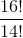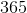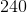Explanation:

Simplify the following factorial:To solve this, we need to understand factorials. Factorials are denoted by a number followed by the ! sign. What a factorial is, is a given number multiplied by each positive integer leading up to the given number. Stated differently...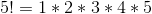So what we have above is really: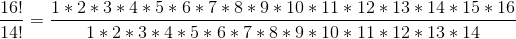Now, because numbers 1 through 14 are in the numerator and the denominator, they will cancel and we will be left with: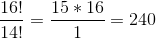### Example Question #18 : Multiplying And Dividing Factorials

Find the value of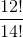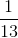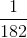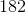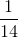Explanation:

If you were to write out the factorial problem above it would look like: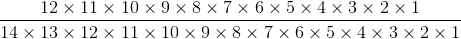The only numbers that do not cancel out in the equation are the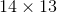in the denominator. Therefore the answer is.

### All Algebra II Resources